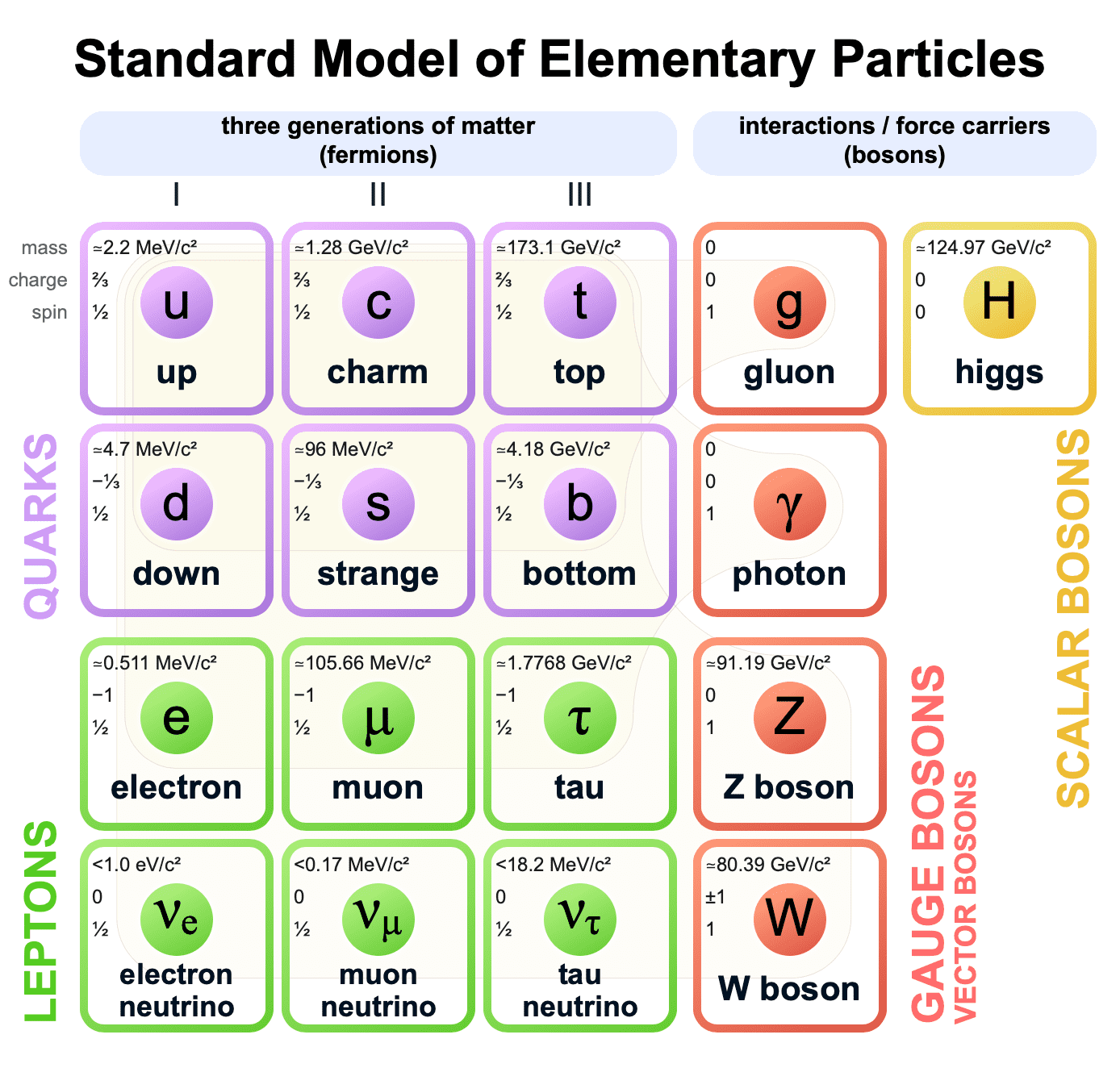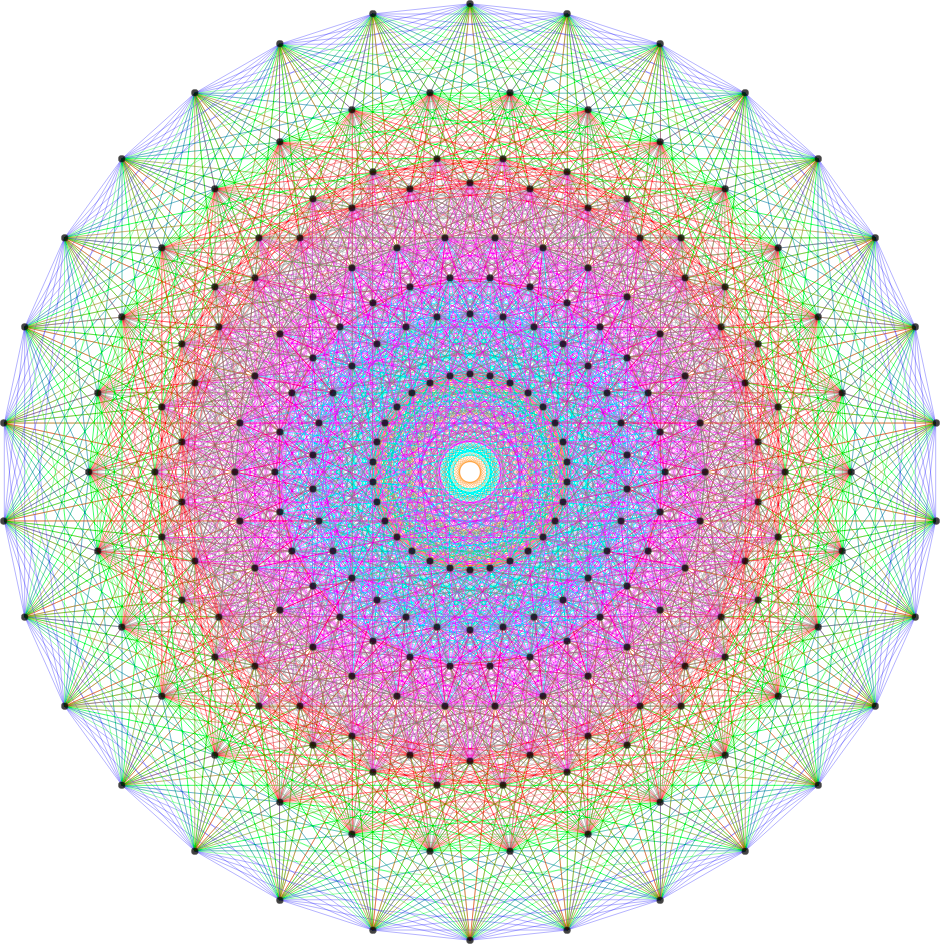# Physics, Reality & The Simulation

1462 words## 18-Dimensional Cellular Automata + E8 Machine

Everything is made of atoms. Protons and neutrons are made of up-quarks and down-quarks. So then everything is made of electrons and up/down-quarks. That’s the fundamental reality.

Actually that’s a lie. The particles are not fundamental. Modern physics says there are no particles, really, there are just fields. The particles are just waves in the fields, they are excitations of fields – the particles do not exist as “primitives” within the system.

What is a field? Mathematically, a field is something that takes a value at every point in space – they aren’t really “made” of anything from a strict physics point of view. Fields permeate all space, including vacuums. Heisenberg’s uncertainty principle says that all fields are constantly in flux – the values for the points in space are always changing and fluctuating. This is why particles are said to “pop in and out of existence” even in a total vacuum. Fields permeate all space, including vacuums, and the fields are always oscillating, which is observed as particles being created/destroyed.

Excitations of just four of the fields are visible – electromagnetic, electron, up-quark, down-quark. But there is a field for each fundamental particle. There are 17 fundamental particles in the Standard Model, so there are at least 17 fields. Space-time is also thought to be a field with a corresponding “graviton” particle, but this has not yet been incorporated into Quantum Field Theory. If the graviton is found then we will have 18 fields.### Gravity, Decay, Anti-Particles

For a long time gravity seemed to imply “action at a distance”. It seemed like mass could affect mass at great distance without any force carrying medium. But General Relativity showed that gravity actually warps the space-time field, and that gravity “waves” travel at the speed of light – so no “action at a distance” required. This is consistent with the field theory, provided the graviton can be found.

Particle decay can be explained by the field theory as well. For example, a neutron can decay into a proton, electron, and anti-neutrino. Neutrons consist of 2 down-quarks, and 1 up-quark. When the decay happens energy simply shifts across fields – from the down-quark field into the up-quark, electron, and anti-neutrino field.

Particles and anti-particles are excitations of the same field, but they are equal and opposite – like a positive number wave of the “normal” particle and a negative number wave for the anti-particle. Because they are equal and opposite waves, they annihilate when they meet.

### Quantum Field Theory

But quantum mechanics is all about discrete particles. So how does that work with continuous fields? This is resolved in Quantum Field Theory. Excitations in the various fields happen only in specific chunks. Those chunks are specified by the mass of the particle for that field – which is shown at the top of each particle cell below. The mass of the particle is the energy needed to vibrate/excite the field. Energy is equivalent to mass by $E=mc^2$, so the particle mass tells us how much energy is needed to create particles on the field.A field will not accept energy below a certain threshold (the particle mass). This discrete unit of energy that the field can accept is called the rest mass energy of the particle. The electron field, for example, requires at least 0.511 MeV to excite the field and create an electron. So the fields are quantized in that they accept energy in specific steps, or units, which we observe as their particles.

### Schrödinger Equation

How are waves in the fields related to the probability waves of quantum mechanics?

We know that it’s wrong to say that an electron is a particle with a position and velocity. It’s not that we can’t measure position and velocity, it’s that these don’t exist. All there is, is a wave function.

Consider a photon travelling through space. That photon exists as a wave in the electromagnetic field. So there is this electromagnetic field, which has a number for each point in space, and this photon represents a moving change in those numbers that make up the field.

One way this could work would be that the number for a single unit of space changes at each time step. Something like the diagram shown below, except in 3-dimensions, where the 1 represents the photon moving “downward” in space.

0 0 1 0 0    0 0 0 0 0    0 0 0 0 0    0 0 0 0 0    0 0 0 0 0
0 0 0 0 0    0 0 1 0 0    0 0 0 0 0    0 0 0 0 0    0 0 0 0 0
0 0 0 0 0 -> 0 0 0 0 0 -> 0 0 1 0 0 -> 0 0 0 0 0 -> 0 0 0 0 0
0 0 0 0 0    0 0 0 0 0    0 0 0 0 0    0 0 1 0 0    0 0 0 0 0
0 0 0 0 0    0 0 0 0 0    0 0 0 0 0    0 0 0 0 0    0 0 1 0 0

In reality, as far as I understand, the photon is a wave spread out over multiple units of space, and the shape of the wave is described by the Schrödinger wave function, so the picture is more like that shown below vs. the one above.

0 0 1 0 0    0 0 0 0 0    0 0 0 0 0    0 0 0 0 0    0 0 0 0 0
0 1 2 1 0    0 0 1 0 0    0 0 0 0 0    0 0 0 0 0    0 0 0 0 0
0 0 1 0 0 -> 0 1 2 1 0 -> 0 0 1 0 0 -> 0 0 0 0 0 -> 0 0 0 0 0
0 0 0 0 0    0 0 1 0 0    0 1 2 1 0    0 0 1 0 0    0 0 0 0 0
0 0 0 0 0    0 0 0 0 0    0 0 1 0 0    0 1 2 1 0    0 0 1 0 0

The wave equation describes, among other things, what the probability will be of “finding the photon” at any given point when measured. Measuring, and interfering with the wave collapses it into a discrete position and momentum. Here is the Schrödinger equation:

$i\hbar {\frac {d}{dt}}\vert \Psi (t)\rangle ={\hat {H}}\vert \Psi (t)\rangle$

### Matrix of Computational 18-tuples

If the universe is all fields, and each field is basically just a number for each point in space, then it would seem the universe could be implemented with something like 18 numbers, or variables, for each point in space, along with the rules for how those values affect their neighbors.

We also know that space is quantized, that its minimum resolution is the Planck length. So, then for a finite region of space you could implement everything with a finite set of 18-tuples. If time is also quantized (it is not known whether this is the case) then the whole thing could be implemented with a finite set of numbers, which all change each time step according to some simple rules.

Basically the whole universe could be a cellular automaton – like Conway’s Game of Life, but in higher dimension. The 18-tuples for every point in space constantly change and oscillate, changes propagate from one cell to another via “direct contact”, simple rules are followed for how the number in one cell affects it’s neighbors, etc. If time is quantized, then the maximum propagation speed (the speed of light) could simply be “one cellular unit propagation per time step”. Then nothing can travel faster than light because to do so that information would have to skip over some units of space.

We know that vast complexity can emerge from cellular automaton operating under simple rules, and can even be Turing-complete. So, it seems that a huge cellular automaton could produce everything we see and experience.

### The E8 Lie GroupThe E8 lie group is a 248 dimensional object, which can also (apparently) be thought of as an object with 8 spacial dimensions and 248 symmetries. Garrett Lisi proposed in his “Exceptionally Simple Theory of Everything”, or “E8 Theory”, that this structure could be the basis for a theory of everything.

So, the E8 group has 248 points, or intersections. According to the Standard Model there are 4 fundamental forces: EM, strong/weak nuclear, and gravity – each with an associated field and particle (assuming the graviton is found). Then there are the 12 non-force-carrying fields and their associated particles. Each of these 12 fundamental particles has an anti-particle. So that makes 24 fundamental particles, plus the 4 force carrying particles, which sums to 28 total particles. Each of these particles has 8 quantum numbers assigned to them based on the charge the particle has. 28 * 8 = 224.

Lisi associated all of these 224 states to a specific point on the E8 group. But, there are 248 points on the E8 object, so Lisi hypothesized 24 yet undiscovered particles. Lisi found that the structure of E8 with the particles assigned to points actually corresponds to observed particle behavior in physics. For example, when photons interact with leptons they create electrons. The same relationship emerges when these particles are mapped onto E8 – the photon point has an edge to the lepton point which has an edge to the electron point.

The E8 structure has a point for the graviton, so the model does predict that the graviton should be found. The model also predicts a 4-dimensional universe, which is obviously what we experience, in contrast to string theory which predicts 10 or 11.

### Matrix of 18-tuples with 248 dimension finite automata

So, finally, and making a lot of assumptions, it would seem that the universe could be implemented with some kind of matrix of 18-tuples for each point in space, combined with this E8 object which acts as the ruleset, defining how those 18-tuples affect each other. Basically, the universe would be implemented as a very large cellular automata with 18 numbers in each cell, and whose rules for how cell values affect their neighbors defined by the E8 structure.